# Important Questions Class 12 Maths Chapter 8-Applications of Integrals

Important For Questions Class 12 Maths Chapter 8 Application of Integrals are provided at BYJU’S along with the detailed explanations. These questions are given as per the new guidelines of CBSE for standard 12th board examination. Students can also practice all the problems of NCERT book along with these questions of Application of Integrals for class 12.

Application of Integrals is the chapter for which students can score good marks after getting thorough with the formulas. We have provided formulas of Class 12 Maths syllabus to help the students revise during their examination. Students can also refer to the important questions for all chapters of Class 12 maths here to prepare well for the CBSE final exam.

## Important Questions & Answers For Class 12 Maths Chapter 8 Application of Integrals

Q. No.1: Find the area enclosed by the ellipse x2/a2 + y2/b2 =1.

Solution:

Given,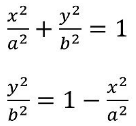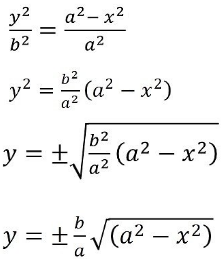We know that,

Ellipse is symmetrical about both x-axis and y-axis.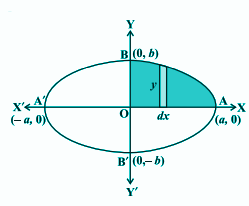Area of ellipse = 4 × Area of AOB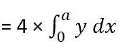Substituting the positive value of y in the above expression since OAB lies in the first quadrant.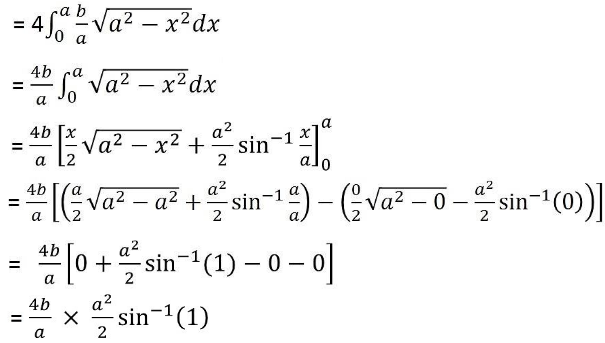= 2ab × sin-1(1)

= 2ab × π/2

= πab

Hence, the required area is πab sq.units.

Q. No. 2: Find the area of the region bounded by y2 = 9x, x = 2, x = 4 and the x-axis in the first quadrant.

Solution:

We can draw the figure of y2 = 9x; x = 2, x = 4 and the x-axis in the first curve as below.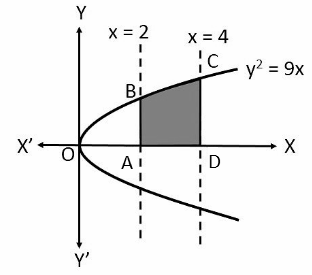y2 = 9x

y = ±√(9x)

y = ±3√x

We can consider the positive value of y since the required area is in the first quadrant.

The required area is the shaded region enclosed by ABCD.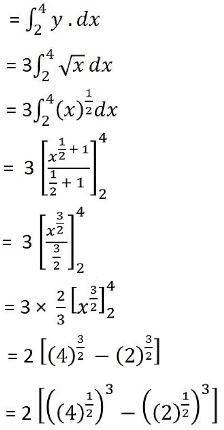= 2 [(2)3 – (√2)3]

= 2[8 – 2√2]

= 16 – 4√2

Hence, the required area is 16 – 4√2 sq.units.

Q. No. 3: Find the area of the curve y = sin x between 0 and π.

Solution:

Given,

y = sin x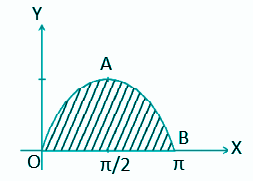Area of OAB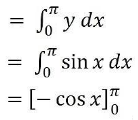= – [cos π – cos 0]

= -(-1 -1)

= 2 sq. units

Q. No. 4: Find the area of the region bounded by the two parabolas y = x2 and y2 = x.

Solution:

Given two parabolas are y = x2 and y2 = x.

The point of intersection of these two parabolas is O (0, 0) and A (1, 1) as shown in the below figure.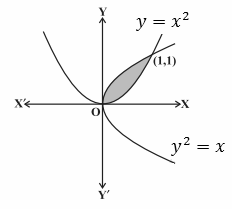Now,

y2 = x

y = √x = f(x)

y = x2 = g(x), where, f (x) ≥ g (x) in [0, 1].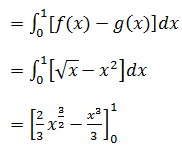= (⅔) – (⅓)

= ⅓

Hence, the required area is ⅓ sq.units.

Q. No. 5: Smaller area enclosed by the circle x2+ y2 = 4 and the line x + y = 2 is

(A) 2 (π – 2)

(B) π – 2

(C) 2π – 1

(D) 2 (π + 2)

Solution:

Option (B) is the correct answer.

Explanation:

Given,

Equation of circle is x2+ y2 = 4……….(i)

x2+ y2 = 22

y = √(22 – x2) …………(ii)

Equation of a lines is x + y = 2 ………(iii)

y = 2 – x

 x 0 2 y 2 0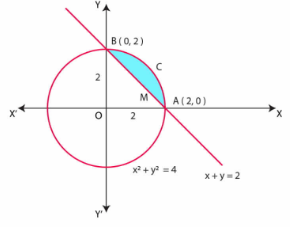Therefore, the graph of equation (iii) is the straight line joining the points (0, 2) and (2, 0).

From the graph of a circle (i) and straight-line (iii), it is clear that points of intersections of circle

(i) and the straight line (iii) is A (2, 0) and B (0.2).

Area of OACB, bounded by the circle and the coordinate axes is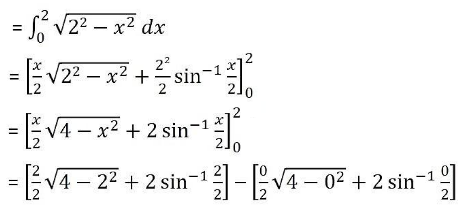= [ 1 × √0 + 2 sin-1(1) – 0√4 – 2 × 0]

= 2 sin-1(1)

= 2 × π/2

= π sq. units

Area of triangle OAB, bounded by the straight line and the coordinate axes is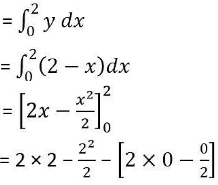= 4 – 2 – 0 + 0

= 2 sq.units

Hence, the required area = Area of OACB – Area of triangle OAB

= (π – 2) sq.units

### Practice Questions For Class 12 Maths Chapter 8 Application of Integrals

1. Find the area of the region bounded by y =√a and y = a.
2. Find the area bounded by the curve y = 4 – x2 and the lines y = 0 and y = 3.
3. Find the area including between the parabolas y2 = 4ax and x2 = 4by.
4. What is the area bounded by the parabola y2 = 8x and x2 = 8y?
5. Find the area bounded by the parabola y2 = 4ax, latus rectum and the x-axis.
6. Using integration, find the area of the region bounded by the line 2y = 5x + 7, x- axis and the lines x = 2 and x = 8.
7. Find the area of the smaller part of the circle x2 + y2 = a2 cut off by the line x = a/√2.